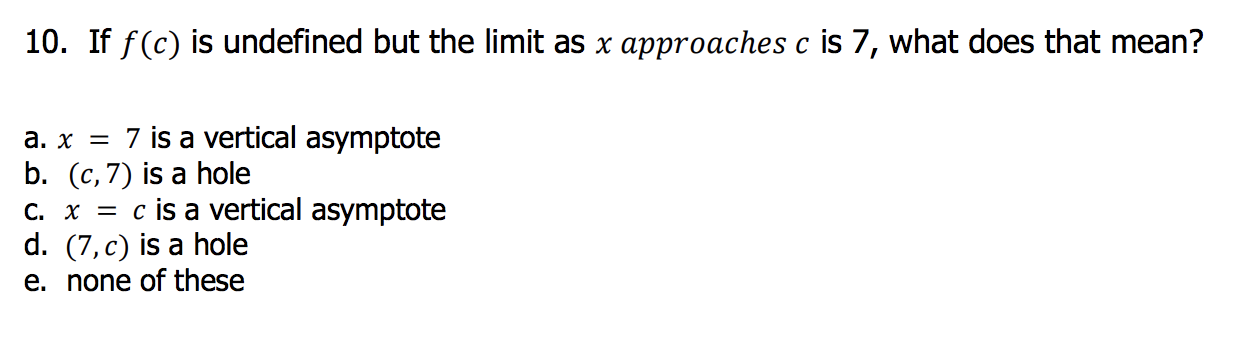# 10. If f(c) is undefined but the limit as x approaches c is 7, what does that mean? a. x7 is a vertical asymptote b. (c,7) is a hole c. x - cis a vertical asymptote d. (7,c) is a hole e. none of these

Questionhelp_outlineImage Transcriptionclose10. If f(c) is undefined but the limit as x approaches c is 7, what does that mean? a. x7 is a vertical asymptote b. (c,7) is a hole c. x - cis a vertical asymptote d. (7,c) is a hole e. none of these fullscreen